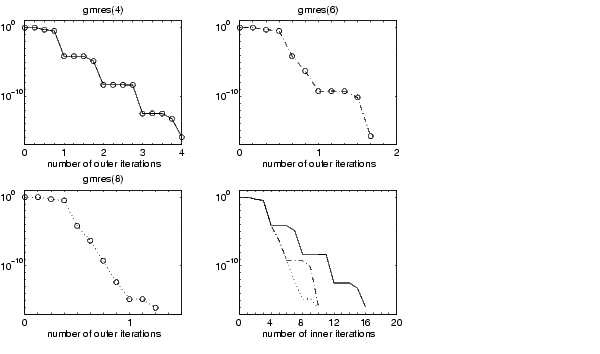MATLAB Function Referencegmres

Generalized Minimum Residual method (with restarts)

Syntax

• ```x = gmres(A,b)
gmres(A,b,restart)
gmres(A,b,restart,tol)
gmres(A,b,restart,tol,maxit)
gmres(A,b,restart,tol,maxit,M)
gmres(A,b,restart,tol,maxit,M1,M2)
gmres(A,b,restart,tol,maxit,M1,M2,x0)
[x,flag] = gmres(A,b,...)
[x,flag,relres] = gmres(A,b,...)
[x,flag,relres,iter] = gmres(A,b,...)
[x,flag,relres,iter,resvec] = gmres(A,b,...)
```

Description

```x = gmres(A,b) ``` attempts to solve the system of linear equations `A*x = b` for `x`. The `n`-by-`n` coefficient matrix `A` must be square and should be large and sparse. The column vector `b` must have length `n`. `A` can be a function handle `afun` such that `afun(x)` returns `A*x`. See Function Handles in the MATLAB Programming documentation for more information.For this syntax, `gmres` does not restart; the maximum number of iterations is `min(n,10)`.

Parameterizing Functions Called by Function Functions, in the MATLAB Mathematics documentation, explains how to provide additional parameters to the function `afun`, as well as the preconditioner function `mfun` described below, if necessary.

If `gmres` converges, a message to that effect is displayed. If `gmres` fails to converge after the maximum number of iterations or halts for any reason, a warning message is printed displaying the relative residual `norm(b-A*x)/norm(b)` and the iteration number at which the method stopped or failed.

```gmres(A,b,restart) ``` restarts the method every `restart` inner iterations. The maximum number of outer iterations is `min(n/restart,10)`. The maximum number of total iterations is `restart*min(n/restart,10)`. If `restart` is `n` or `[]`, then `gmres` does not restart and the maximum number of total iterations is `min(n,10)`.

```gmres(A,b,restart,tol) ``` specifies the tolerance of the method. If `tol` is `[]`, then `gmres` uses the default, `1e-6`.

```gmres(A,b,restart,tol,maxit) ``` specifies the maximum number of outer iterations, i.e., the total number of iterations does not exceed `restart*maxit`. If `maxit` is `[]` then `gmres` uses the default, `min(n/restart,10)`. If `restart` is `n` or `[]`, then the maximum number of total iterations is `maxit` (instead of `restart*maxit`).

```gmres(A,b,restart,tol,maxit,M) and gmres(A,b,restart,tol,maxit,M1,M2) ``` use preconditioner `M` or `M = M1*M2` and effectively solve the system `inv(M)*A*x = inv(M)*b` for `x`. If `M` is `[]` then `gmres` applies no preconditioner. `M` can be a function handle `mfun` such that `mfun(x)` returns `M\x`.

```gmres(A,b,restart,tol,maxit,M1,M2,x0) ``` specifies the first initial guess. If `x0` is `[]`, then `gmres` uses the default, an all-zero vector.

```[x,flag] = gmres(A,b,...) ``` also returns a convergence flag:

 `flag = 0` `gmres` converged to the desired tolerance `tol` within `maxit` outer iterations. `flag = 1` `gmres` iterated `maxit` times but did not converge. `flag = 2` Preconditioner `M` was ill-conditioned. `flag = 3` `gmres` stagnated. (Two consecutive iterates were the same.)

Whenever `flag` is not `0`, the solution `x` returned is that with minimal norm residual computed over all the iterations. No messages are displayed if the `flag` output is specified.

```[x,flag,relres] = gmres(A,b,...) ``` also returns the relative residual `norm(b-A*x)/norm(b)`. If `flag` is `0`, `relres <= tol`.

```[x,flag,relres,iter] = gmres(A,b,...) ``` also returns both the outer and inner iteration numbers at which `x` was computed, where `0 <= iter(1) <= maxit` and `0 <= iter(2) <= restart`.

```[x,flag,relres,iter,resvec] = gmres(A,b,...) ``` also returns a vector of the residual norms at each inner iteration, including `norm(b-A*x0)`.

Examples

Example 1.

• ```A = gallery('wilk',21);
b = sum(A,2);
tol = 1e-12;
maxit = 15;
M1 = diag([10:-1:1 1 1:10]);

x = gmres(A,b,10,tol,maxit,M1);
```

displays the following message:

• ```gmres(10) converged at outer iteration 2 (inner iteration 9) to
a solution with relative residual 3.3e-013
```

Example 2.

This example replaces the matrix `A` in Example 1 with a handle to a matrix-vector product function `afun`, and the preconditioner `M1` with a handle to a backsolve function `mfun`. The example is contained in an M-file `run_gmres` that

• Calls `gmres` with the function handle `@afun` as its first argument.
• Contains `afun` and `mfun` as nested functions, so that all variables in `run_gmres` are available to `afun` and `mfun`.

The following shows the code for `run_gmres`:

• ```function x1 = run_gmres
n = 21;
A = gallery('wilk',n);
b = sum(A,2);
tol = 1e-12;  maxit = 15;
x1 = gmres(@afun,b,10,tol,maxit,@mfun);

function y = afun(x)
y = [0; x(1:n-1)] + ...
[((n-1)/2:-1:0)'; (1:(n-1)/2)'].*x + ...
[x(2:n); 0];
end

function y = mfun(r)
y = r ./ [((n-1)/2:-1:1)'; 1; (1:(n-1)/2)'];
end
end
```

When you enter

• ```x1 = run_gmres;
```

MATLAB displays the message

• ```gmres(10) converged at outer iteration 2 (inner iteration 9) to
a solution with relative residual 3.3e-013
```

Example 3.

• ```load west0479
A = west0479
b = sum(A,2)
[x,flag] = gmres(A,b,5)
```

`flag `is `1` because `gmres` does not converge to the default tolerance `1e-6` within the default 10 outer iterations.

• ```[L1,U1] = luinc(A,1e-5);
[x1,flag1] = gmres(A,b,5,1e-6,5,L1,U1);
```

`flag1` is` 2` because the upper triangular `U1` has a zero on its diagonal, and `gmres` fails in the first iteration when it tries to solve a system such as `U1*y = r` for `y` using backslash.

• ```[L2,U2] = luinc(A,1e-6);
tol = 1e-15;
[x4,flag4,relres4,iter4,resvec4] = gmres(A,b,4,tol,5,L2,U2);
[x6,flag6,relres6,iter6,resvec6] = gmres(A,b,6,tol,3,L2,U2);
[x8,flag8,relres8,iter8,resvec8] = gmres(A,b,8,tol,3,L2,U2);
```

`flag4`, `flag6`, and `flag8` are all `0` because `gmres` converged when restarted at iterations 4, 6, and 8 while preconditioned by the incomplete LU factorization with a drop tolerance of `1e-6`. This is verified by the plots of outer iteration number against relative residual. A combined plot of all three clearly shows the restarting at iterations 4 and 6. The total number of iterations computed may be more for lower values of `restart`, but the number of length `n` vectors stored is fewer, and the amount of work done in the method decreases proportionally.See Also

`bicg`, `bicgstab`, `cgs`, `lsqr`, `luinc`, `minres`, `pcg`, `qmr`, `symmlq`

`function_handle` (`@`), `mldivide` (`\`)

References

  Barrett, R., M. Berry, T. F. Chan, et al., Templates for the Solution of Linear Systems: Building Blocks for Iterative Methods, SIAM, Philadelphia, 1994.

  Saad, Youcef and Martin H. Schultz, "GMRES: A generalized minimal residual algorithm for solving nonsymmetric linear systems", SIAM J. Sci. Stat. Comput., July 1986, Vol. 7, No. 3, pp. 856-869.

© 1994-2005 The MathWorks, Inc.